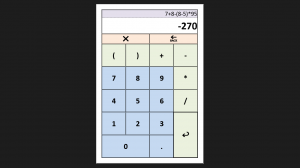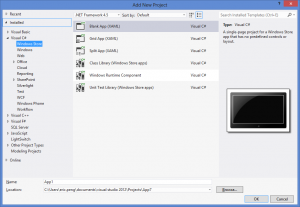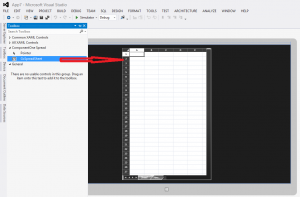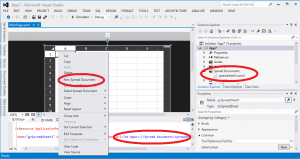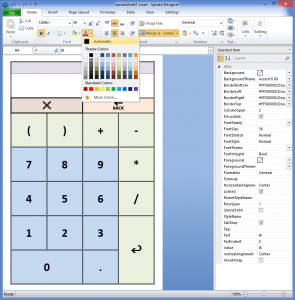```void gcSpreadSheet1_CellClick(object sender, CellClickEventArgs e)
{
if (e.Column == 4 && e.Row == 7) //=
{
sheet[2, 1].Formula = "";
try
{
sheet[2, 1].Formula = sheet[1, 1].Text;
}
catch
{
sheet[2, 1].Text = "Invalid Formula!";
}
}
else if (e.Column == 1 && e.Row == 3) //C
{
sheet[1, 1].ResetText();
sheet[2, 1].ResetText();
sheet[2, 1].Formula = "";
}
else if (e.Column == 3 && e.Row == 3) //BS
{
var txt = sheet[1, 1].Text;
if (txt == string.Empty) return;
sheet[1, 1].Text = txt.Substring(0, txt.Length - 1);
}
else if (e.Column > 0 && e.Column < 5 && e.Row > 3 && e.Row < 9) //digits and signs
{
sheet[1, 1].Text = sheet[1, 1].Text + sheet[e.Row, e.Column].Text;
sheet[2, 1].ResetText();
sheet[2, 1].Formula = "";
}
}```